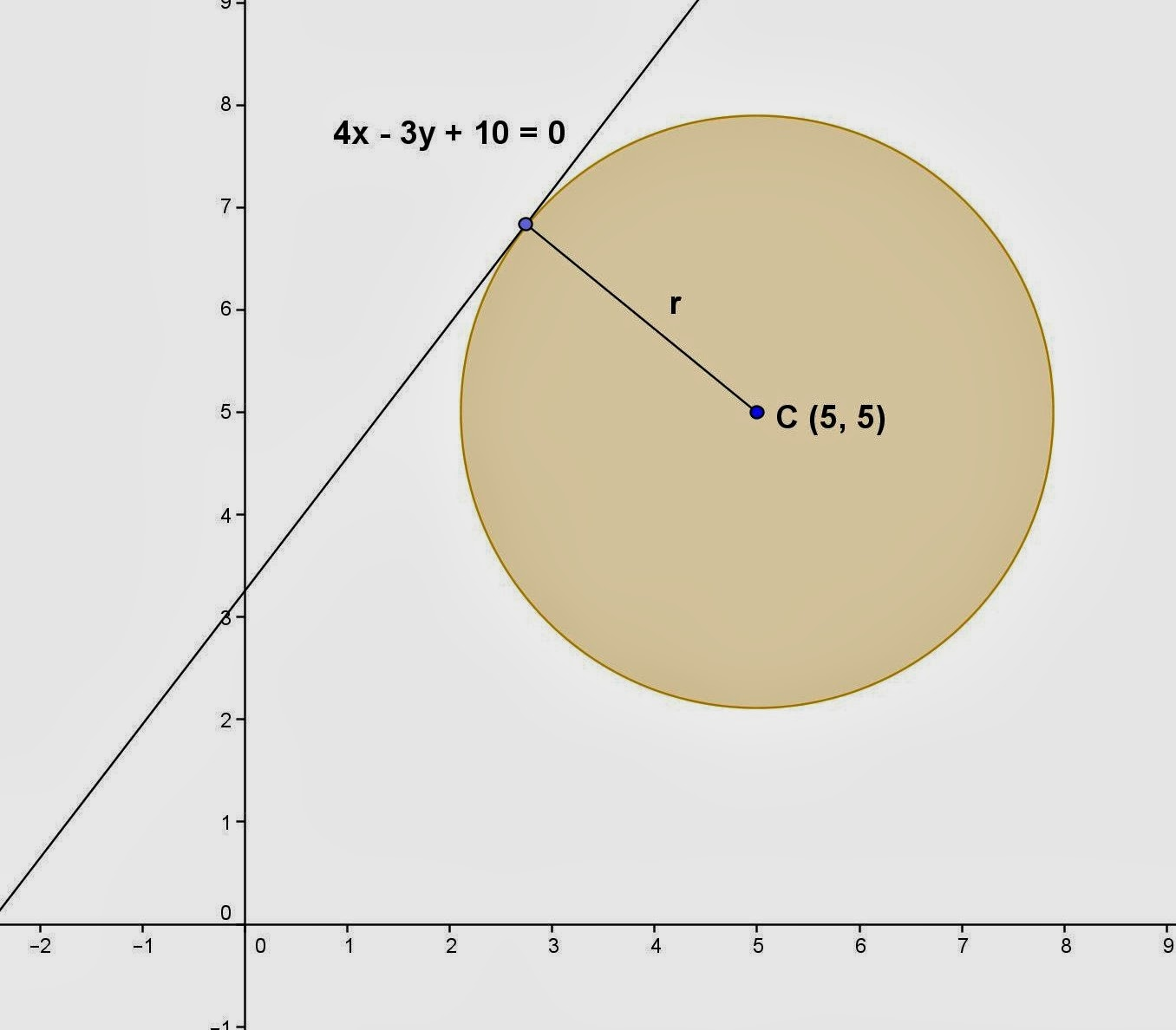Saturday, January 25, 2014

Finding Equation - Circle, 4

Category: Analytic Geometry, Plane Geometry

"Published in Newark, California, USA"

Find the equation of a circle that is tangent to the line 4x - 3y + 10 = 0 and its center at (5, 5).

Solution:

To illustrate the problem, it is better to draw the figure as followsPhoto by Math Principles in Everyday Life

The radius of a circle can be solved by using the perpendicular distance of a point to the line formula as follows

where A, B, and C are the coefficients of the equation of a given line. Hence, the above equation becomes

Since the coefficient of 10 is positive, then it follows that the sign of a radical is negative. Take the opposite sign of 10.

Substitute the values of x and y from the coordinates of a center of a circle, we have

The radius of a circle is always a positive value. We need to take the absolute value for that one. Hence, r = 3.

Therefore, the equation of a circle is